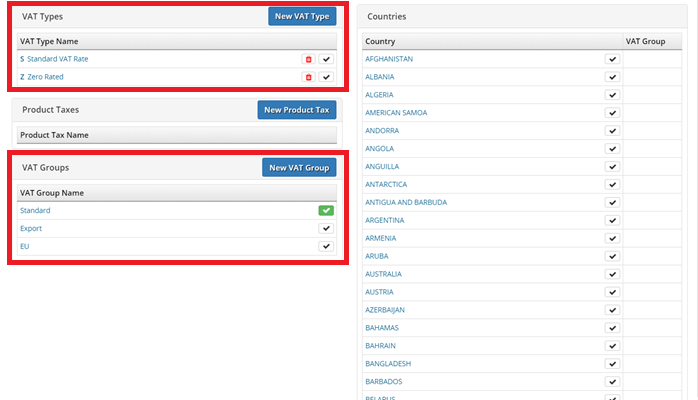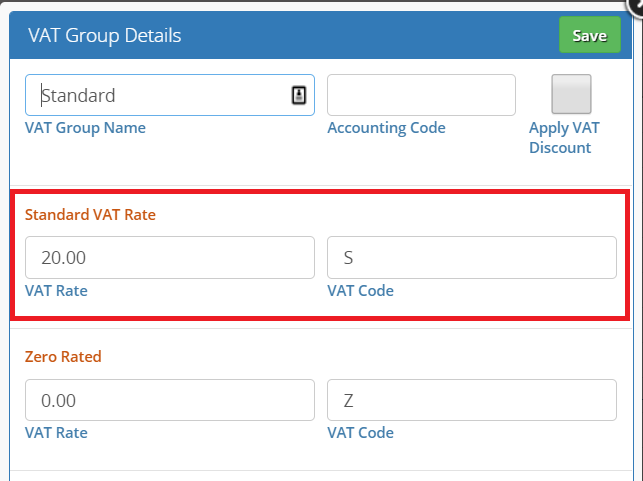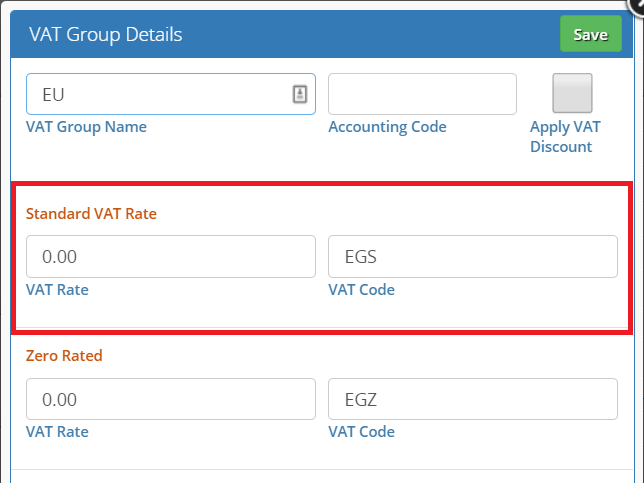The VAT type and group are used together to determine the actual VAT rate for a given item on a given order.
An order will have a VAT group assigned to it, and each line in the order will have a VAT type set for it. Each VAT group contains settings for each VAT type. So, we use both to determine the VAT rate.Above is the setting for the ‘Standard’ VAT group. It lists all the VAT types, and, in each type, you set the VAT rate. In this case, the Standard VAT group defines the Standard VAT type’s rate as 20%, and the Zero VAT type’s rate as 0%.
Contrast this to the EU VAT group. In that VAT group we can see that Standard VAT type’s rate is 0% and the VAT code is different too.
VAT Type
Define VAT types here and use them in item settings to set the items VAT type.
VAT Group
Define the VAT groups here, and then use them in a Sales Order